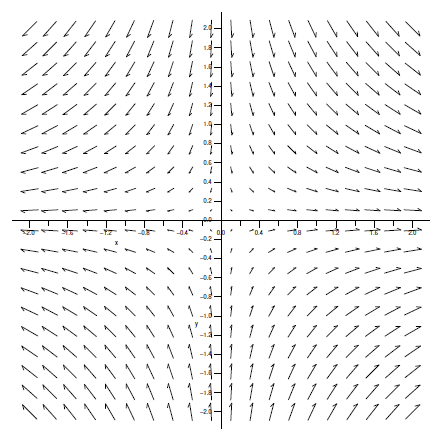$$\newcommand{\id}{\mathrm{id}}$$ $$\newcommand{\Span}{\mathrm{span}}$$ $$\newcommand{\kernel}{\mathrm{null}\,}$$ $$\newcommand{\range}{\mathrm{range}\,}$$ $$\newcommand{\RealPart}{\mathrm{Re}}$$ $$\newcommand{\ImaginaryPart}{\mathrm{Im}}$$ $$\newcommand{\Argument}{\mathrm{Arg}}$$ $$\newcommand{\norm}{\| #1 \|}$$ $$\newcommand{\inner}{\langle #1, #2 \rangle}$$ $$\newcommand{\Span}{\mathrm{span}}$$

16.1: Vector Fields

•• Contributed by David Guichard
• Professor (Mathematics) at Whitman College

$$\newcommand{\vecs}{\overset { \rightharpoonup} {\mathbf{#1}} }$$

$$\newcommand{\vecd}{\overset{-\!-\!\rightharpoonup}{\vphantom{a}\smash {#1}}}$$

This chapter is concerned with applying calculus in the context of vector fields. A two-dimensional vector field is a function $$\vecs{f}$$ that maps each point $$(x,y)$$ in $$\mathbb{R}^2$$ to a two-dimensional vector $$\langle u,v\rangle$$, and similarly a three-dimensional vector field maps $$(x,y,z)$$ to $$\langle u,v,w\rangle$$. Since a vector has no position, we typically indicate a vector field in graphical form by placing the vector $$\vecs{f}(x,y)$$ with its tail at $$(x,y)$$. Figure $$\PageIndex{1}$$ shows a representation of the vector field

$\vecs{f}(x,y)=\langle -x/\sqrt{x^2+y^2+4},y/\sqrt{x^2+y^2+4}\rangle.$

For such a graph to be readable, the vectors must be fairly short,which is accomplished by using a different scale for the vectors than for the axes. Such graphs are thus useful for understanding the sizes of the vectors relative to each other but not their absolute size.Figure $$\PageIndex{1}$$. A vector field.

Vector fields have many important applications, as they can be used to represent many physical quantities: the vector at a point may represent the strength of some force (gravity, electricity, magnetism) or a velocity (wind speed or the velocity of some other fluid).

We have already seen a particularly important kind of vector field---the gradient. Given a function $$f(x,y)$$, recall that the gradient is $$\langle f_x(x,y),f_y(x,y)\rangle$$, a vector that depends on (is a function of) $$x$$ and $$y$$. We usually picture the gradient vector with its tail at $$(x,y)$$, pointing in the direction of maximum increase. Vector fields that are gradients have some particularly nice properties, as we will see. An important example is

$$\vecs{F}=\left \langle {-x\over (x^2+y^2+z^2)^{3/2}},{-y\over (x^2+y^2+z^2)^{3/2}},{-z\over (x^2+y^2+z^2)^{3/2}}\right\rangle,$$

which points from the point $$(x,y,z)$$ toward the origin and has length

$$|| \vecs{F}||= {\sqrt{x^2+y^2+z^2}\over(x^2+y^2+z^2)^{3/2}} {1\over(\sqrt{x^2+y^2+z^2})^2},$$

which is the reciprocal of the square of the distance from $$(x,y,z)$$ to the origin---in other words, $$\vecs{F}$$ is an "inverse square law''. The vector $$\vecs{F}$$ is a gradient:

$\vecs{F} = \nabla \dfrac{1}{\sqrt{x^2+y^2+z^2}}$

which turns out to be extremely useful.

Contributors

• Integrated by Justin Marshall.